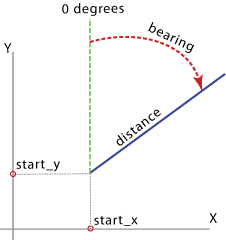# Bearing Distance To Line (Data Management)

## Summary

Creates a feature class containing geodetic line features constructed based on the values in an x-coordinate field, y-coordinate field, bearing field, and distance field of a table.

## Illustration## Usage

• Each geodetic line is constructed using a particular set of field values representing the x and y coordinates of a starting point, distance from the starting point, and bearing angle measured from North. These fields and values will be included in the output.

• A geodetic line is a curve on the surface of the earth. However, a geodetic line feature is not stored as a parametric (true) curve in the output; rather, it is stored as a densified polyline representing the path of the geodetic line. If the length of a geodetic line is relatively short, it may be represented by a straight line in the output. As the length of the line increases, more vertices are used to represent the path.

• When the output is a feature class in a geodatabase, the values in the Shape_Length field are always in the units of the output coordinate system specified by the Spatial Reference parameter, and they are the planar lengths of the polylines. To measure a geodesic length or distance, use the ArcGIS Pro Measure tool and choose the Geodesic, Loxodrome, or Great Elliptic option accordingly before taking a measurement.

## Syntax

`arcpy.management.BearingDistanceToLine(in_table, out_featureclass, x_field, y_field, distance_field, {distance_units}, bearing_field, {bearing_units}, {line_type}, {id_field}, {spatial_reference}, {attributes})`
 Parameter Explanation Data Type in_table The input table. It can be a text file, CSV file, Excel file, dBASE table, or geodatabase table. Table View out_featureclass The output feature class containing densified geodetic lines. Feature Class x_field A numerical field in the input table containing the x-coordinates (or longitudes) of the starting points of lines to be positioned in the output coordinate system specified by the spatial_reference parameter. Field y_field A numerical field in the input table containing the y-coordinates (or latitudes) of the starting points of lines to be positioned in the output coordinate system specified by the spatial_reference parameter. Field distance_field A numerical field in the input table containing the distances from the starting points for creating the output lines. Field distance_units(Optional) Specifies the units for the distance_field parameter.METERS —The distance unit is meters.KILOMETERS —The distance unit is kilometers.MILES —The distance unit is miles.NAUTICAL_MILES —The distance unit is nautical miles.FEET —The distance unit is feet.US_SURVEY_FEET —The distance unit is U.S. Survey feet. String bearing_field A numerical field in the input table containing bearing angle values for the output line rotation. The angles are measured clockwise from North. Field bearing_units(Optional) Specifies the units of the bearing_field parameter values. DEGREES — The units will be decimal degrees. This is the default.MILS —The units will be mils.RADS —The units will be radians.GRADS —The units will be gradians. String line_type(Optional) Specifies the type of geodetic line to construct.GEODESIC — A type of geodetic line that most accurately represents the shortest distance between any two points on the surface of the earth will be constructed. The mathematical definition of the geodesic line is quite lengthy and complex and is therefore omitted here. This is the default.GREAT_CIRCLE —A type of geodetic line that represents the path between any two points along the intersection of the surface of the earth and a plane that passes through the center of the earth will be constructed. Depending on the output coordinate system specified by the Spatial Reference parameter, in a spheroid-based coordinate system, the line is a great elliptic; in a sphere-based coordinate system, the line is uniquely called a great circle—a circle of the largest radius on the spherical surface.RHUMB_LINE —A type of geodetic line, also known as a loxodrome line, that represents a path between any two points on the surface of a spheroid defined by a constant azimuth from a pole will be constructed. A rhumb line is shown as a straight line in the Mercator projection.NORMAL_SECTION —A type of geodetic line that represents a path between any two points on the surface of a spheroid defined by the intersection of the spheroid surface and a plane that passes through the two points and is normal (perpendicular) to the spheroid surface at the starting point of the two points will be constructed. Therefore, the normal section line from point A to point B is different from the one from point B to point A. String id_field(Optional) A field in the input table. This field and the values are included in the output and can be used to join the output features with the records in the input table. Field spatial_reference(Optional) The spatial reference of the output feature class. A spatial reference can be specified as any of the following: The path to a .prj file, such as C:/workspace/watershed.prj The path to a feature class or feature dataset whose spatial reference you want to apply, such as C:/workspace/myproject.gdb/landuse/grassland A SpatialReference object, such as arcpy.SpatialReference("C:/data/Africa/Carthage.prj") Spatial Reference attributes(Optional) Specifies whether the remaining input fields will be written to the output feature class.NO_ATTRIBUTES —The remaining input fields will not be written to the output feature class. This is the default.ATTRIBUTES —The remaining input fields will be included in the output feature class. A new field, ORIG_FID, will also be added to the output feature class to store the input feature ID values. Boolean

## Code sample

BearingDistanceToLine example (stand-alone script)

Convert bearing and distance info into a line.

``````# Import system modules
import arcpy

# Local variables
input_table = r'c:\workspace\LOBtraffic.dbf'
output_fc = r'c:\workspace\SOPA.gdb\lob_traf001'

# BearingDistanceToLine
arcpy.BearingDistanceToLine_management(input_table, output_fc, 'X', 'Y',
'NAUTICAL_MILES', 'azim', 'DEGREES',
'GEODESIC', 'recnum')``````

## Licensing information

• Basic: Yes
• Standard: Yes